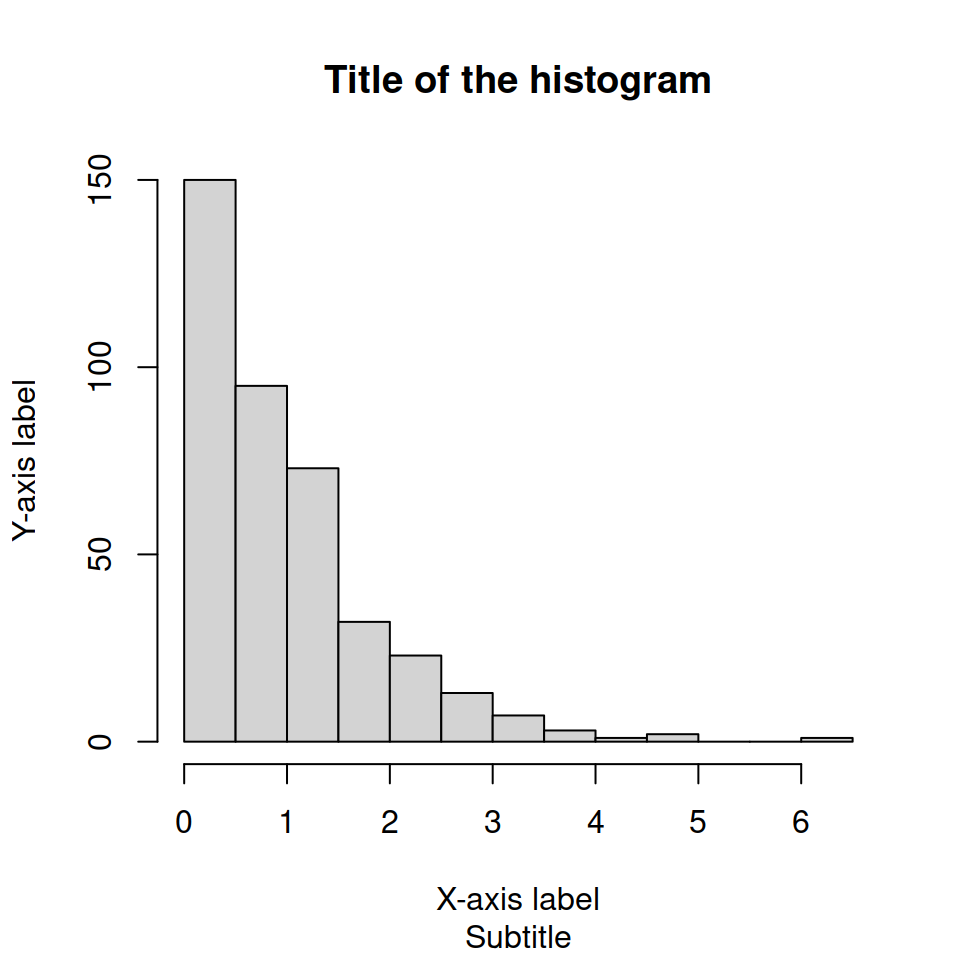# Frequency histogram in R

## A basic frequency histogram

The `hist` function allows creating histograms in base R. By default, the function will create a frequency histogram.

``````# Sample data (exponential)
set.seed(1)
x <- rexp(400)

# Histogram
hist(x)``````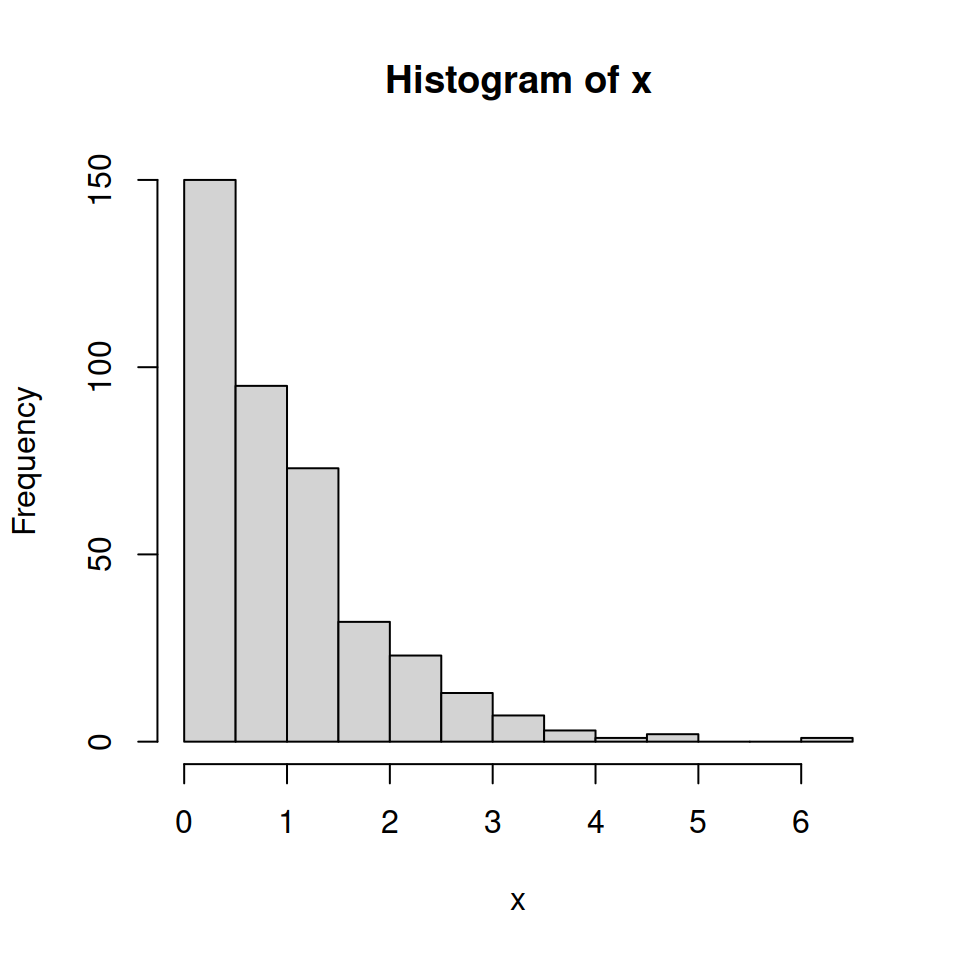## Color of the histogram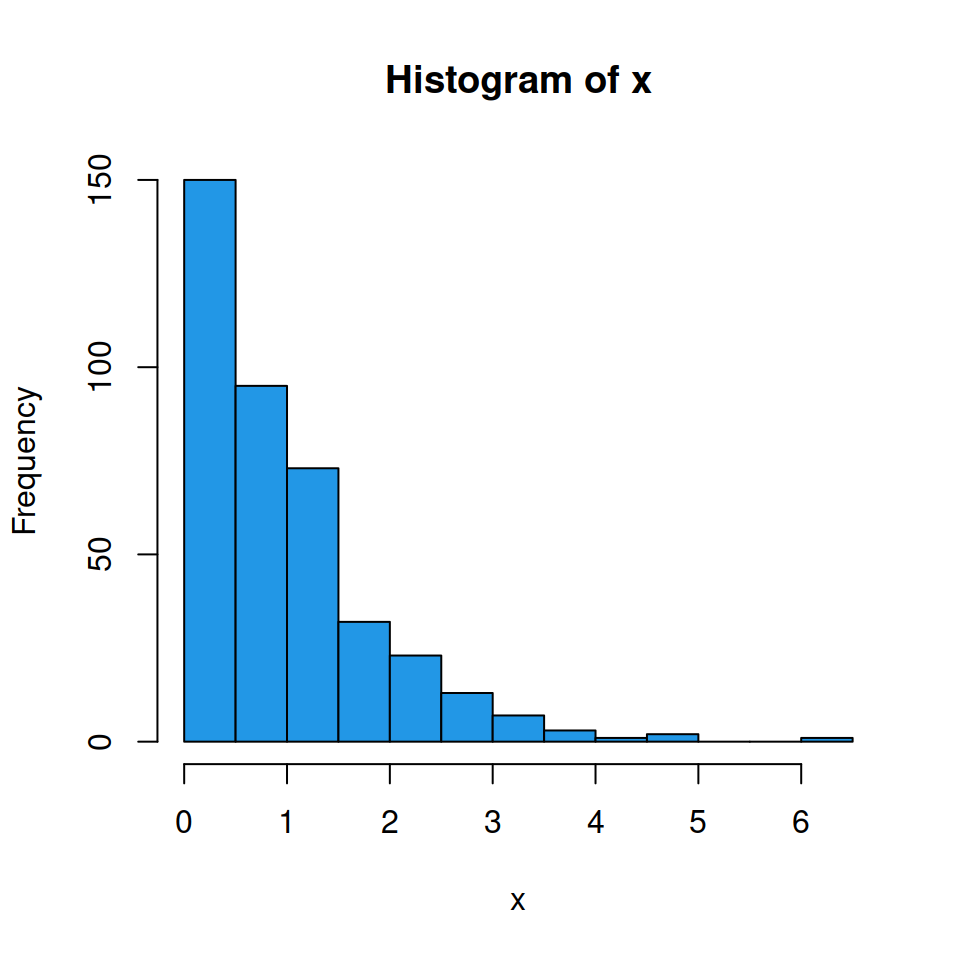Fill color

You can change the default fill color of the histogram bars (gray since R 4.0.0) to other making use of the `col` argument.

``````# Sample data (exponential)
set.seed(1)
x <- rexp(400)

# Blue histogram
hist(x,       # Data
col = 4) # Color``````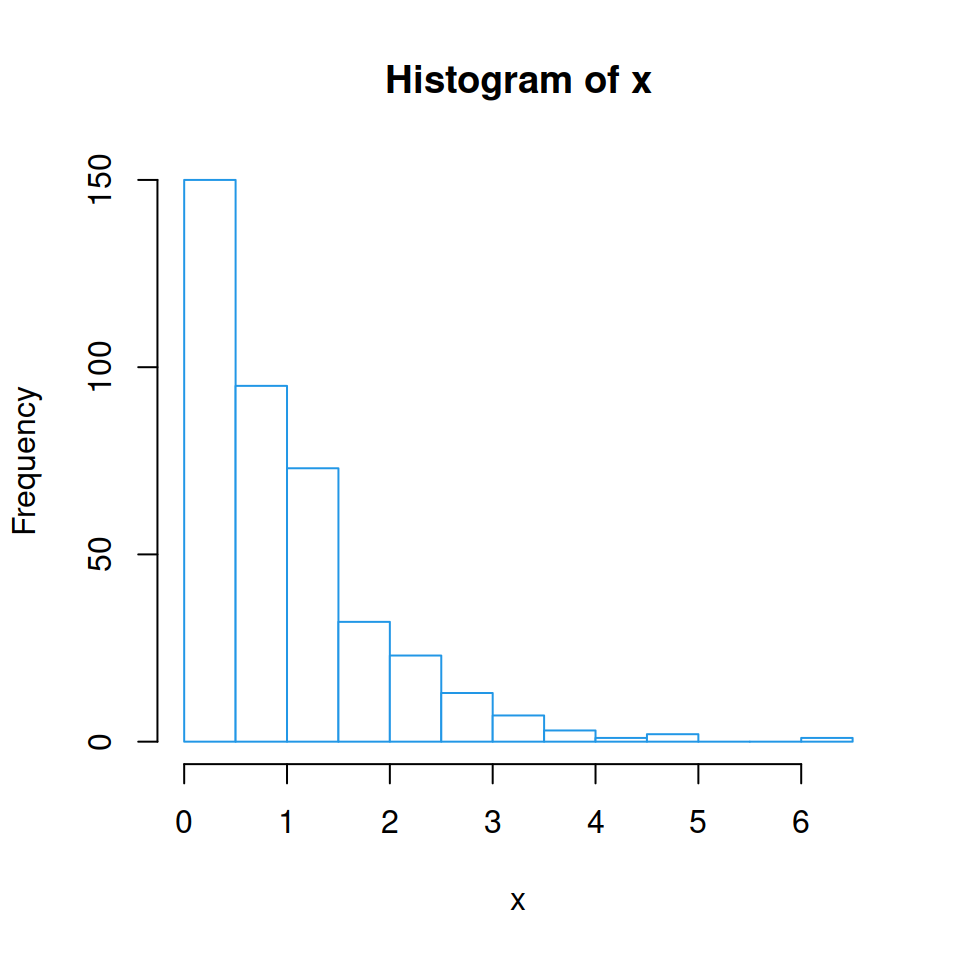Border color

The `border` argument allows modifying the border color of the bars.

``````# Sample data (exponential)
set.seed(1)
x <- rexp(400)

# White histogram with blue borders
hist(x,
col = "white", # Fill color
border = 4)    # Border``````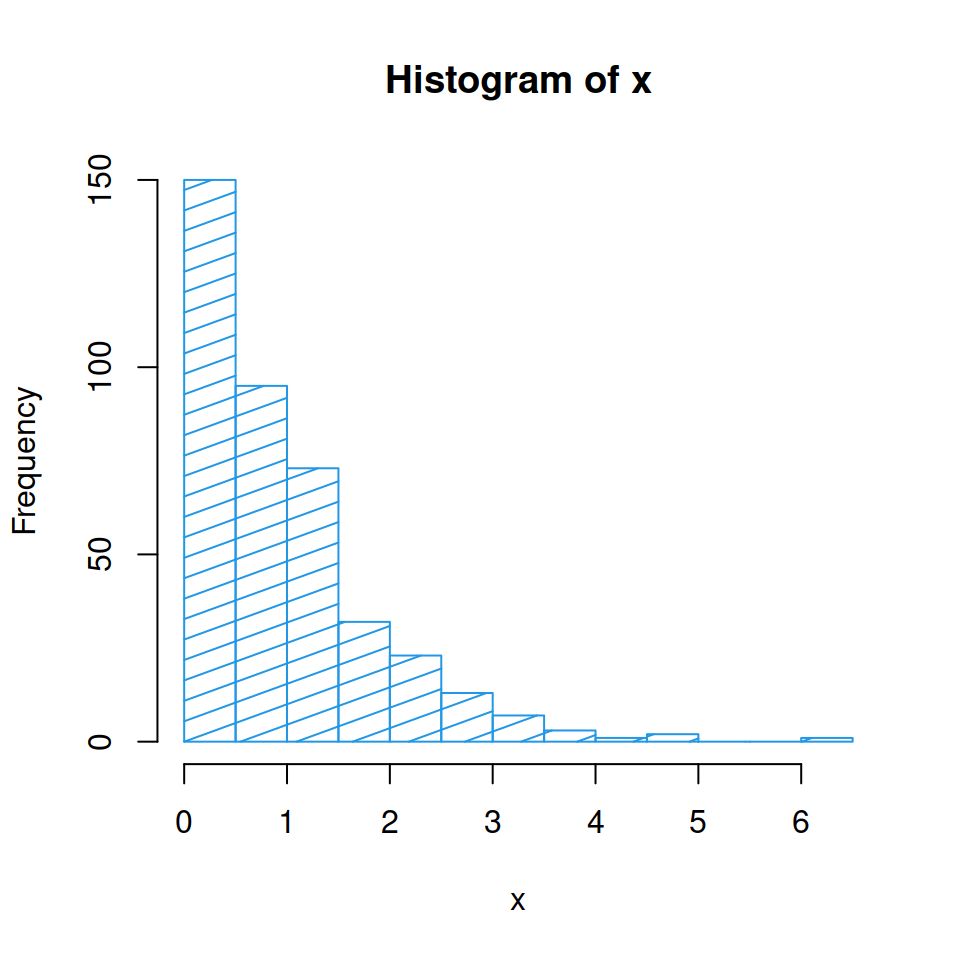You can also use shading lines instead of a fill color. Set them with the `density` argument and modify its angle with `angle`.

``````# Sample data (exponential)
set.seed(1)
x <- rexp(400)

# White histogram with shading lines
hist(x,
col = 4,      # Color
density = 10, # Shading lines
angle = 20)   # Shading lines angle``````

## Titles and labels

You can also modify the title, subtitle, and axes labels with `main`, `sub`, `xlab` and `ylab` arguments, respectively.

``````# Sample data (exponential)
set.seed(1)
x <- rexp(400)

# Histogram with titles
hist(x,
main = "Title of the histogram", # Title
sub = "Subtitle",                # Subtitle
xlab = "X-axis label",           # X-axis label
ylab = "Y-axis label")           # Y-axis label``````